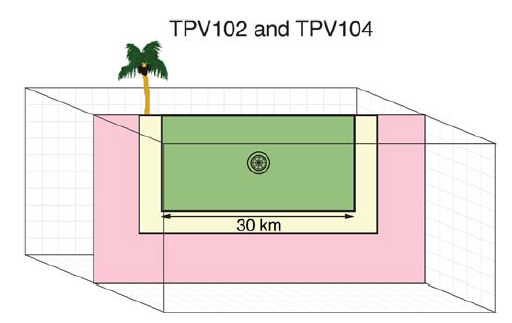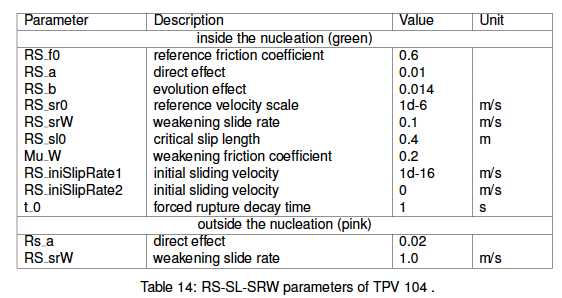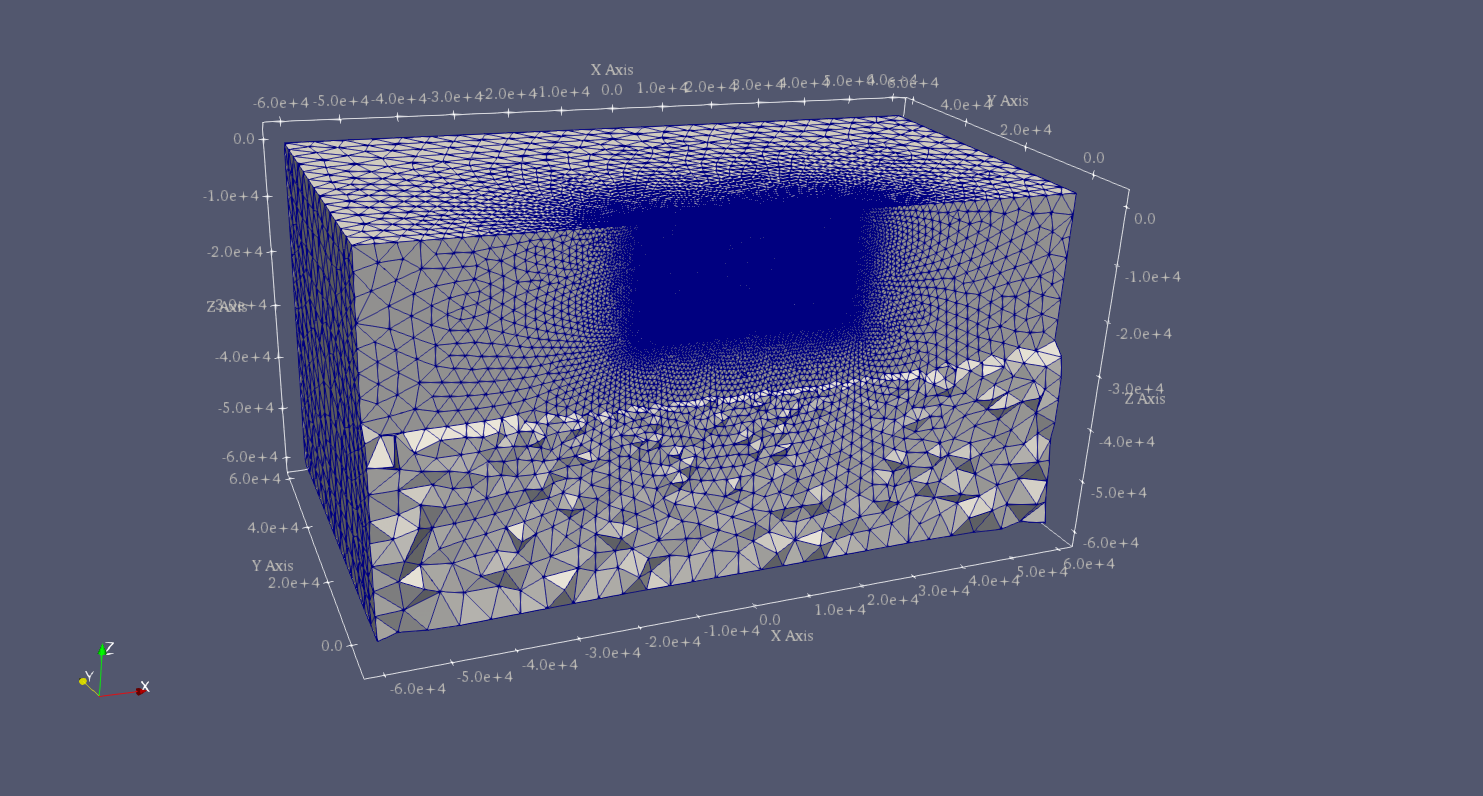# SCEC TPV104¶

In this example, we illustrate how to implement rate-state friction law using a slip law with strong rate weakening (RS-SL-SRW) and setup parameters in SeisSol.

TPV104 has a planar rectangular vertical strike-slip fault with the main rupture region of velocity-weakening friction, a zone on the fault surface with transitional friction surrounds the main fault rupture region, and the outer regions on the fault surface have velocity-strengthening friction (Figure [fig:tpv104]).Diagram of TPV104. The vertical planar fault that has velocity-weakening friction region (green), which is surrounded by velocity-strengthening region (pink). It encounters a finite width transitional region (yellow) where the friction smoothly changes from velocity weakening (green) on the inside to velocity strengthening (red) on the outside.

## Geometry¶

TPV104 uses the same vertical fault as TPV5. We use the mesh file of TPV5 directly.

## RSL parameters¶

TPV104 uses rate-state friction where shear stress follows:

\begin{aligned} \tau = f(V,\psi) \sigma\end{aligned}

The friction coefficient is a function of slip rate $$V$$ and state $$\psi$$:

\begin{aligned} f(V,\psi) = a * arcsinh [\frac{V}{2V_0} \exp(\frac{\psi}{a})]\end{aligned}

The state variable evolves according to the equation:

\begin{align}\begin{aligned} \begin{aligned} \frac{d \psi}{dt} = - \frac{V}{L}[\psi - \psi_{ss}(V)]\end{aligned}\\and\end{aligned}\end{align}
\begin{aligned} \psi_{ss}(V) = a \ln [\frac{2V_0}{V} \sinh (\frac{f_{ss}(V)}{a})]\end{aligned}

$$f_{ss}(V)$$ is the stead state friction coefficient that depends on $$V$$ and the friction parameters $$f_0, V_0, a, b, f_w and V_w$$.

\begin{aligned} f_{ss}(V) = f_w + \frac{f_{LV}(V) - f_w}{[1+(V/V_w)^8]^{1/8}}\end{aligned}

with a low-velocity steady state friction coefficient:

\begin{aligned} f_{LV}(V) = f_0 + (b-a) * \ln (V/V_0)\end{aligned}

In SeisSol input file, Rate-state friction law can be used by choosing FL=103 in parameter.par. The friction parameters of RS-SL-SRW are shown in Table [table:tpv104rsl].Table of rate-state friction used in tpv104.

To stop the rupture, the friction law changes from velocity-weakening in the rectangular interior region of the fault to velocity-strengthening sufficiently far outside this region. The transition occurs smoothly within a transition layer of width w = 3 km. Outside the transition layer, the fault is made velocity-strengthening by increasing $$a$$ by $$\triangle a= 0.01$$ and $$V_w$$ by $$\triangle V_{w0} = 0.9$$ .

The input files of TPV104 can be found at https://github.com/SeisSol/Examples/tree/master/tpv104.Diagram shows the tetrahedral meshing of TPV 104 shown in the ParaView panel.

## Results¶

The earthquake nucleates in the velocity-weakening zone spontaneously. The rupture propagates through the transition zone into the velocity-strengthening region, where it smoothly and spontaneously arrests. Nucleation is done by imposing additional shear stress in a circular patch surrounding the hypocenter.

Figure [fig:tpv104sr] shows the slip rate on the fault along the downdip direction at T=5s.Slip rate along-strike on the fault at 2 s of TPV104.Slip rate along-strike on the fault at 5 s of TPV 104.